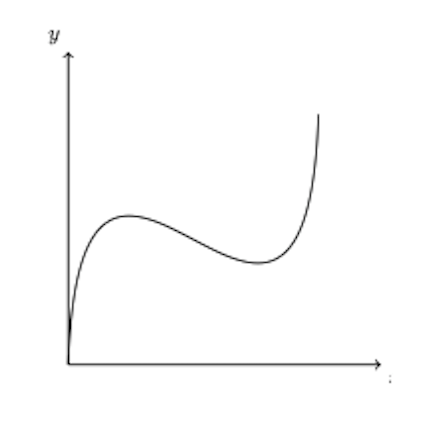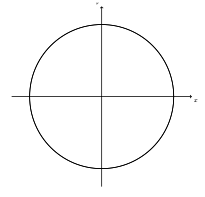Is This Relation a Function?

This post about whether a relation is a function is part of a series of posts to help you prepare for the Advanced Algebra and Functions part of the Accuplacer test.

Question

One or more of the graphs below represent 𝑦 as a function of 𝑥. Which one or ones?ABCD

Solution

Function/not function?

A function is a relation – that is, a correspondence between x and  y – for which every x-value relates to only one y-value. No vertical line will pass through more than one point on a function’s graph, but a vertical line may pass through more than one point on the graph of a relation that is not a function. Thus you can use the vertical line test on a relation to see whether it is a function.

Look at relation A. Imagine sliding a vertical line across it. At no point will the line intersect the relation more than once. This relation is a function.

Now look at relation B. The y-axis, which is a vertical line, intersects the relation in three places. Thus relation B fails the vertical line test and relation B is not a function.

Relation C: Again, there are places where you could draw a vertical line and cross the relation more than once. For example, the y-axis crosses the relation twice. This relation is not a function.

Relation D: There is only one x-value where it looks like a vertical line could cross this relation twice: the x-value at the right-hand end of the lower piece, which is the x-value at left-hand end of the upper piece. Are there two y-values at this x-value? The lower piece, in which the circle is filled in, does take this x-value. But the upper piece, in which the circle is not filled in, does not take the value. Thus there is no x-value that both pieces take, and there is no x-value for which there is more than one value of  This relation is a function.

𝑨𝒏𝒔𝒘𝒆𝒓: A and D

0 replies